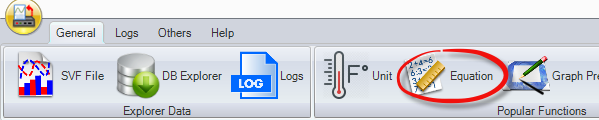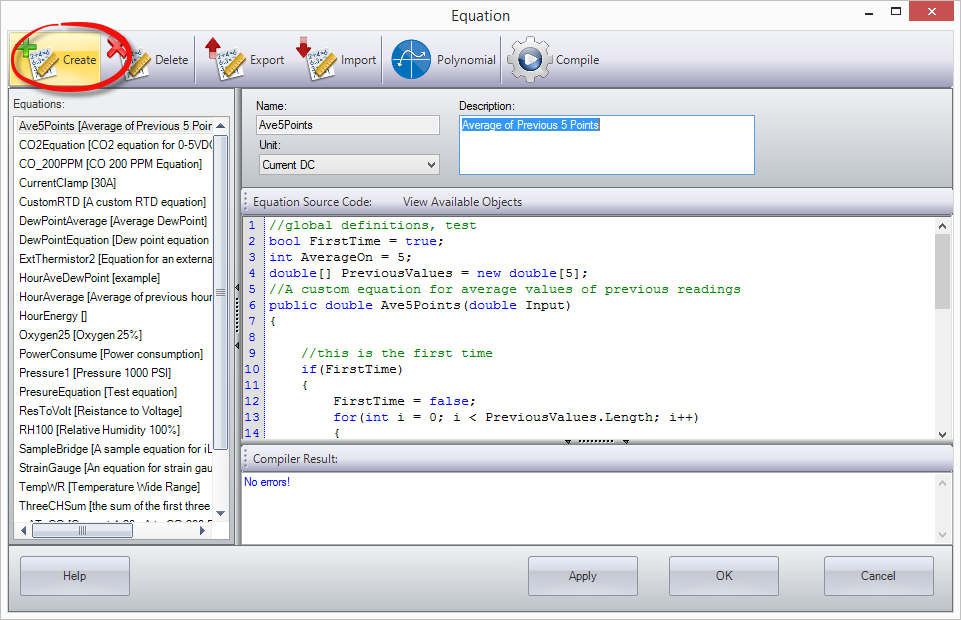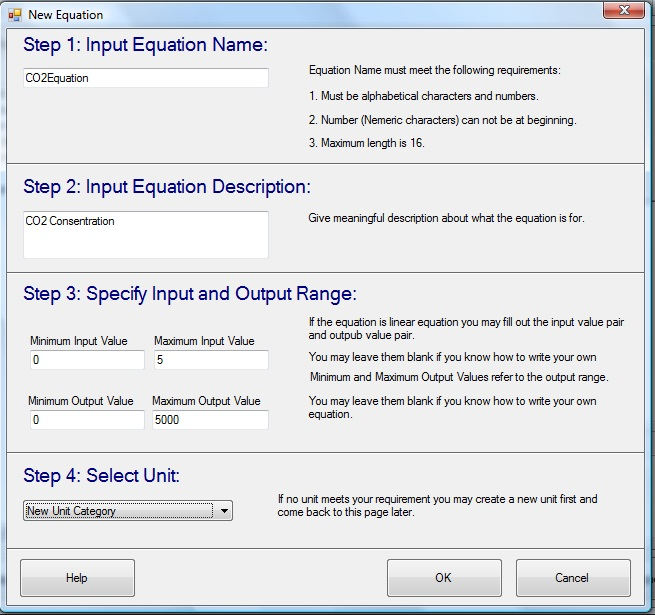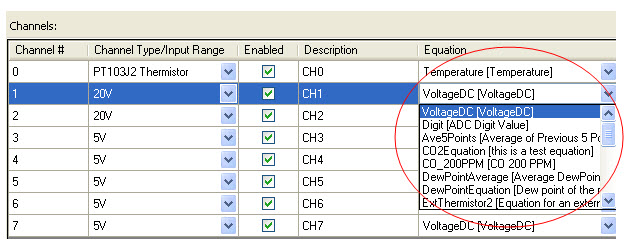# What is a custom equation and when can it be used?

A custom equation is a snippet of script (C# source code) does the data conversion for a custom measurement. You use custom equation if your measurement unit is different from the unit of measurement the channel provides.

To create a custom equation:
• Click “Equation” button on the main tool bar:• In the equation dialog, click “Create” button to create a new equation:• In the pop-up dialog, enter the name and description of the equationIf the equation is a linear equation, type in the Input Value pair and Output Value pair. In the above example, the correct parameters are:

Minimum Input Value = 0
Maximum Input Value = 10

Minimum Output Value = 0
Maximum Output Value = 5000

You need to choose a unit for the new equation. If this is a new unit you need to use Unit Dialog to create a unit prior to this dialog.
• Click OK button to close the dialog and return to the Equation dialog.

The new equation is added to the equation list on the left-hand side and the contents of the equation are displayed on the right-hand side panel.

• Click Apply button to save the new created equation.

To use a custom equation in a channel:

Open Logger Configuration dialog. When you click on the combo-list in the Equation column cell, all custom equations will be displayed in the list.  Choose the one and click Apply button to save the equation information to the logger.